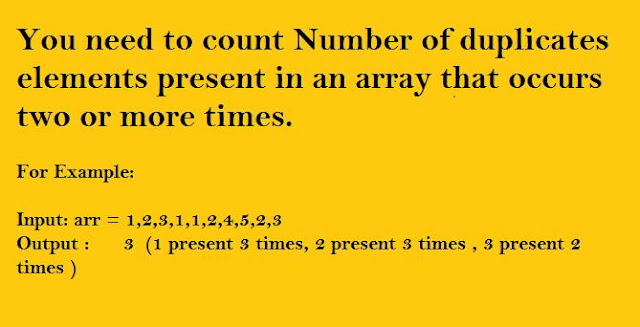# Count Number Of Duplicates Elements Present In Array

Count Number Of Duplicates Elements Present In Array

You need to count Number of duplicates elements present in an array that occurs two or more times.

For Example:

Input: arr = 1,2,3,1,1,2,4,5,2,3
Output :      3  (1 present 3 times, 2 present 3 times , 3 present 2 times )Count-Duplicates-In-Array

#include<iostream>
#include<stdio.h>
using namespace std;
int main()
{
int arr[] = { 1,2,3,1,1,4,5,6,4,4,5,2,2,12};
int size1 = sizeof(arr)/sizeof(arr);
int count1 = 0,flag=0, temp = 0;;
int n = 0;

/*first sort array elements so that all duplicates will be next to each other*/

for (int i = 0; i<size1; i++)
{
for (int j = 0; j<size1 - i - 1; j++)
{
if (arr[j] > arr[j + 1])
{
temp = arr[j];
arr[j] = arr[j + 1];
arr[j + 1] = temp;
}
}
}

/*Print sorted array*/

for (int i = 0; i<size1; i++)
cout << arr[i] << "  ";
int i = 0;
while(i<size1)
{
flag = 0;
for (int j = i + 1; j<=size1; j++)
{
if (arr[i] == arr[j])
{
n++;
flag++;
if (flag ==1)
count1++;
continue;
/*if elements present the continue to next iteration inside nested loop*/
}
else
{
n++;
break;
}
}
i = n;
}
cout << "\n\n count value:-  " << count1;
getchar();

}